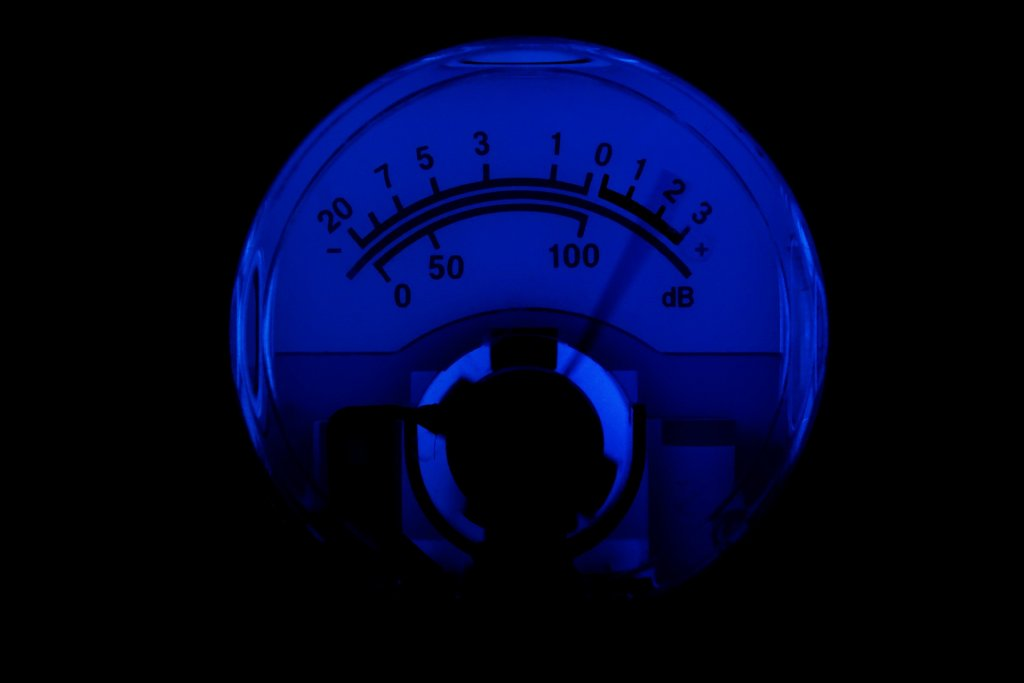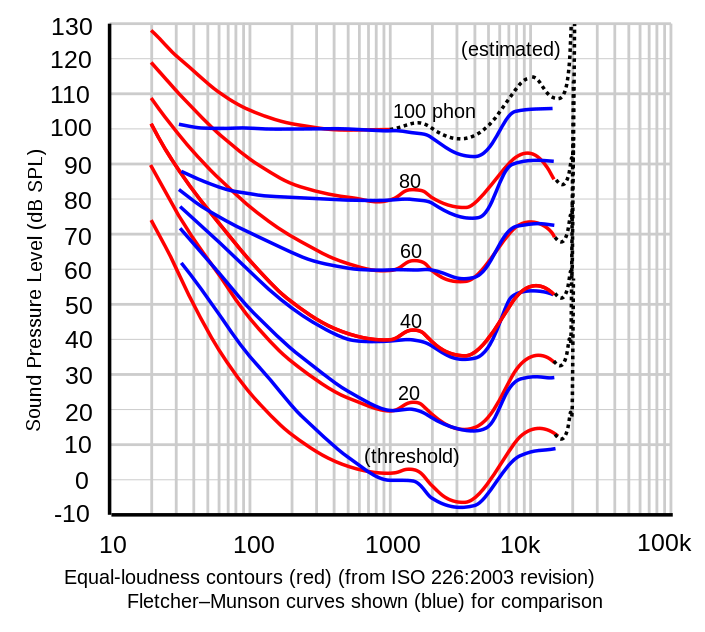Currently viewing Vol. 3 • Issue 2 • 2016

# Can We Measure Our Age in dB? … You Must Be Kidding?No, we are not kidding! But why can’t we measure our age, weight, or height, in decibels?

When we know an age, it is measured in terms of time, generally in years. A year is a unit of time as are hours, minutes, and seconds. But, at no place was there a suggestion to use the dB as a “unit”; just to express something other than sound level.

## A UNIT?

What is a unit? According to Wikipedia (https://en.wikipedia.org/wiki/Decibel), "A unit of measurement is a definite magnitude of a physical quantity, defined and adopted by convention or by law, that is used as a standard for measurement of the same physical quantity."

The decibel is used not only in acoustics, but in electronics, engineering, and in the control theory, having been developed near the beginning of the 20th century by the Bell Telephone system in the USA. But that doesn't make it a unit? According to the above definition, the decibel is not a physical quantity – only a relation.

## A RELATION?

The decibel is indeed merely a relation and not a unit; equal to 20 times the ratio of a quantity divided by another, and depending on what it is divided by it can be used as reference. Both, the quantity and the reference must be measured in the same value with the same dimensions. In the case of sound pressure level (SPL), it is the relation of the measured squared sound pressure divided by the reference pressure of 20*10−6N/m2.

## AND THE AGE … PLAYING WITH dB?

What about our age (or our height or weight)? First, a reference age needs to be assigned − for instance 20 years. Then we ask, what will be the age, in dB, of a person of say, 40 years of age? The result is as follows:

20*log10(40/20) = 6 dB
However, if we change the reference age to his retirement age (65 years), the result changes to

20*log10(40/65) = −4 dB

## WHAT ABOUT THE HEARING THRESHOLD LEVEL?

As we all know, the hearing sensitivity is a function of the frequency of the sound. In 1933, Fletcher and Munson published the first equal-loudness curves for minimal audible field (see Figure 1), where we have a plot of the sound pressure level in dB SPL against the frequency of the tones or other stimuli, used for the determination, in Hz. The 0 phon curve − the lowest of them (named “threshold”) − corresponds to the the lowest level, expressed in dBHL, at which the individual can detect the presence of the stimulus for at least 50% of stimulus presentations. The minimal audible pressure threshold curve (not shown) is the one that used for testing hearing under earphones.Our audiometers are calibrated following the threshold curves, meaning that when we set our instrument to present a tone under earphones of 0 dBHL at 1000 Hz, this is equivalent to saying that we are presenting a tone of 7 dB SPL. One could argue that this difference between dB HL and dB SPL is minimal at 1000 Hz, but if we go further down in frequency, for instance at 250 Hz, our audiometer is generating a sound pressure level of 25.5 dB SPL, and 45.5 dB SPL at 125 Hz.

If a decibel was a unit, there would not be such a difference; but a decibel is merely a relation and can be used to express any number of things such as age, weight, height, and temperature.